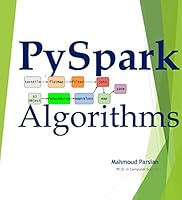PySpark Algorithms0 Reviews
2019-08-16
682 pages

Book Description

This book is about PySpark: Python for Spark. Apache Spark is an analytics engine for large-scale data processing. Spark is the cluster system that makes data analytics fast to write and fast to run. This book provides a large set of recipes for implementing big data processing and analytics using Spark and Python. The goal of this book is to show working examples in PySpark so that you can do your ETL and analytics easier. You may cut and paste examples to deliver your applications in PySpark.

This book introduces PySpark (Python API for Spark). You can use PySpark to tackle big datasets quickly through simple APIs in Python. You will learn how to express tasks and computations with just a few lines of code, and cover applications from ETL, simple batch jobs to stream processing and machine learning.

With this book, you may dive into Spark capabilities such as RDDs (resilient distributed datasets), dataframes (data as a table of rows and columns), in-memory caching, and the interactive PySpark shell, where you may leverage Spark's powerful built-in libraries, including Spark SQL, Spark Streaming, and MLlib.

In this book, you will learn Spark's transformations and actions by a set of well-defined and working examples. All examples are tested and working: this means that you can copy-cut-paste to your desired PySpark applications. PySpark is much easier than Spark applications in Java and PySpark applications are not bulky at all when compared to Java Spark.

In this book you will learn:

• Short introduction to Spark and PySpark
• Learn about RDDs, DataFrames, SQL with worked examples
• How to use important Spark transformations on RDDs (low-level APIs)
• How to use SQL and DataFrame
• How to read data from many different data sources and represent them as RDDs and DataFrames
• Learn the power of Data Design Patterns
• Learn the basics of Monoids and how you should use them in MapReduce
• Learn the basics of GraphFrames for solving graph-related data problems
• Implement Logistic Regression using PySpark
• Basics of data partitioning and understand reduction transformations

Book Details

• Title: PySpark Algorithms
• Author:
• Length: 682 pages
• Edition: 1
• Language: English
• Publication Date: 2019-08-16
• ISBN-10: B07WQHTVCJ